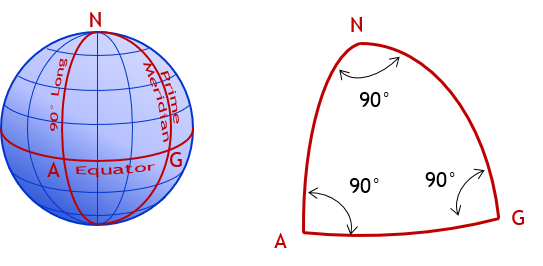## 3. Geodetic Surveying

The physical earth is three dimensional and quite irregular due to mass anomalies, rotational wobble, centrifugal force, lunar attraction, etc. Geodetic Surveying takes into account the size and shape of the earth, Figure C-5.Figure C-5 Geodetic Surveying

Complex mathematical models are used to create a three dimensional datum to approximate the earth and measurements must be transferred from the physical earth to and from the datum. Vertical lines converge making computations more complex. Unlike flat triangles in plane surveying, geodetic triangles are spherical with angle sums exceeding 180°, sometimes by quite a bit. For example, in a triangle created by intersecting the Prime Meridian, 90th meridian, and the Equator, Figure C-6, each angle is 90° for a total of 270°.Σ(angles) = 270°00’00” Figure C-6 Spherical Triangle

In Geodetic Surveying, because the earth is curved, gravity lines converge. A horizontal line is perpendicular to a vertical line at only a single point, Figure C-7.Figure C-7 Vertical Lines in Geodetic Surveying